## Elastic soil-structure interaction – is it worth the effort?

 Author(s) Mick Pender Published 24 November 2017 Collection NZGS Symposium Link N/A Compilation 20th NZGS Symposium
Elastic soil-structure interaction – is it worth the effort?

# Abstract

It is widely acknowledged that soil-structure interaction (SSI) has an important effect on foundation performance during earthquake loading. The standard way of handling this is to assume elastic behaviour of the soil beneath and around the foundation. For shallow foundation response there are three dimensionless parameters which indicate the effect of SSI. These are functions of six properties of the structure-foundation system: the shear wave velocity and density of the foundation soil, the natural frequency of the fixed base structure, the height of the structure, the mass of the structure, and the dimensions of the foundation. However, elastic soil behaviour occurs only at very small shear strains. For realistic foundation proportions the effect of elastic soil-structure-interaction (SSI) is frequently quite small and so the response of the foundation is only marginally different from the fixed base response; the purpose of the paper is demonstrate this. The paper explains that real foundation designs involve larger shear strains than those for elastic behaviour over a substantial volume of soil beneath the foundation and so to achieve enhanced foundation performance from interaction with the soil below it is important to consider nonlinear soil behaviour. Nonlinear soil behaviour is approximated using a reduced shear modulus, the effect of such reduction is discussed.

# 1 INTRODUCTION

It is widely acknowledged that soil-structure interaction (SSI) has an important effect on foundation performance during earthquake loading. The standard way of handling this is to assume elastic behaviour of the soil beneath and around the foundation. Consideration of an earthquake design response spectrum, such as that shown in Figure 1, illustrates how SSI induced period lengthening of the system may reduce structural design actions if the periods concerned are located along the falling branch of the design spectrum.

Wolf (1985) and others have shown that there are three dimensionless parameters which indicate the significance of elastic SSI. These are functions of six properties of the structure-foundation system: the shear wave velocity of the foundation soil, the density of the foundation soil, the natural frequency of the fixed base structure, the mass of the structure, the height of the structure, and the dimensions of the foundation. The dimensionless quantities are the stiffness ratio (SR), the slenderness ratio (HR) and the mass ratio (MR):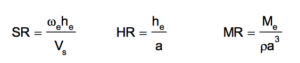(1)

where: Me is the mass of the structure supported on the foundation, a is the radius of a circle having the same area as the foundation, he is the height above foundation level of the centroid of the structural mass, is the density of the soil, Vs is the shear wave velocity of the soil, and s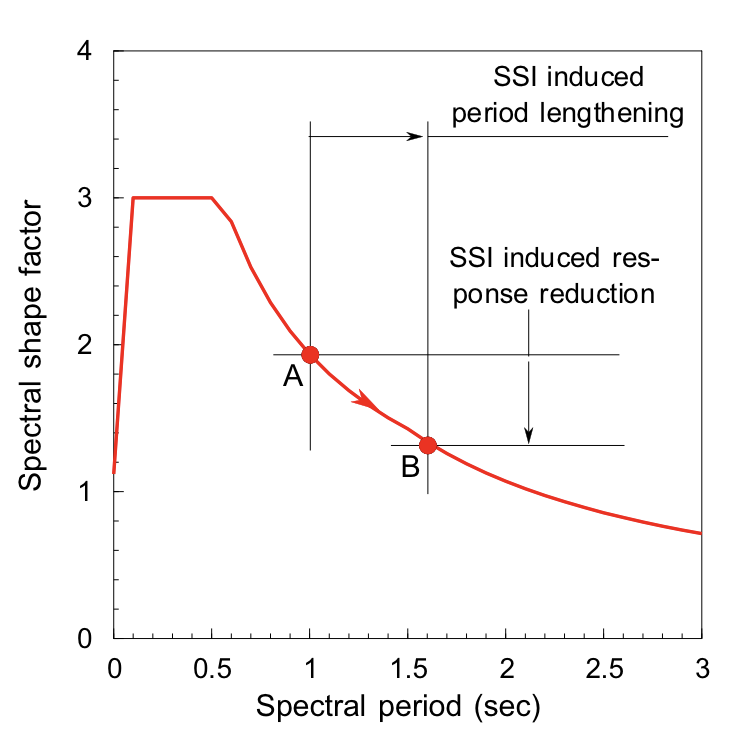Figure 1: Potential beneficial effect of soil-structure interaction when the period of the fixed base structure is located along the falling branch of a design spectrum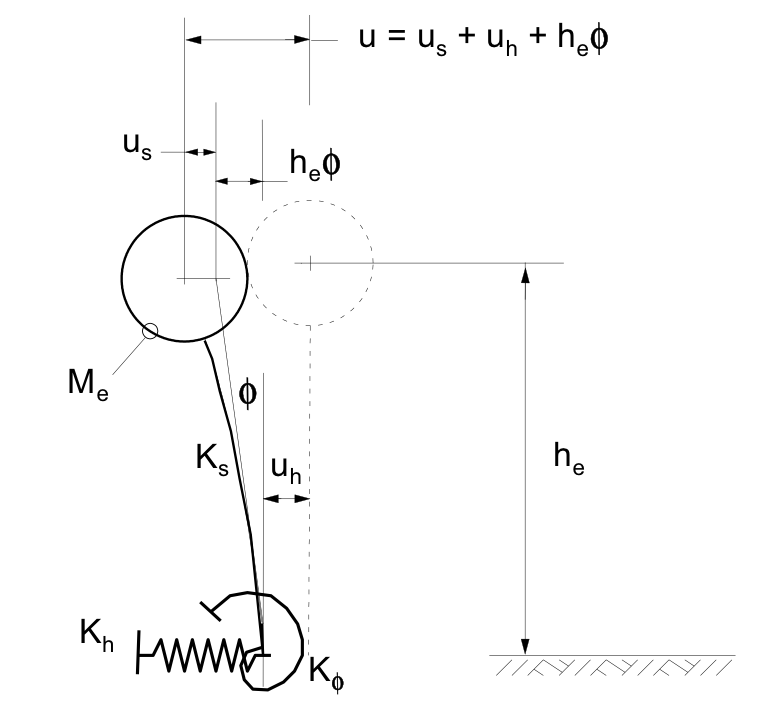Figure 2: Single degree of freedom (SDOF) model of a structure-foundation system with a concentrated mass, Me located he above the foundation, and three springs (Ks, Kh and K)

is the natural frequency (radians/second) of the fixed base structure. Note that the shear wave velocity is a property of the soil at very small (ie elastic) shear strains.

The stiffness ratio compares the elastic stiffness of the fixed base structure to the elastic properties of the foundation soil. The slenderness ratio is a geometric parameter comparing structural and foundation dimensions. The mass ratio can be thought of as the mass of the structure in relation

Table 1: Soil layer properties 4 m beneath the ground surface

 Soil density state Shear wave velocity (Vs) (m/sec) Density () (tonnes/m3) Small strain shear modulus (Go) (MPa) Loose (L) 160 1.70 44 Medium (M) 210 1.85 82 Dense (D) 250 2.00 125

to the mass of a volume of soil beneath the foundation which contributes to the foundation stiffness; as this ratio decreases the inertia of the foundation soil becomes more significant.

Frequently, a single degree of freedom (SDOF) model is used to represent to the first mode response of a structural system. Such a model is shown in Figure 2 in which a concentrated mass Me represents the mass of the structure; a column, height he, represents the lateral stiffness, Ks, of the structure; a spring, stiffness Kh, represents the lateral stiffness of the foundation; and a spring, stiffness K, represents the rotational stiffness of the foundation. Figure 2 shows how the lateral displacement of the mass consists of three components: the bending of the structural column, he times the rotation of the foundation, and the lateral displacement of the foundation. The natural frequency of such a model (readily established with a pushover analysis) is given by: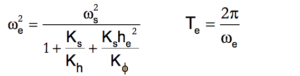(2)

where: e and s are respectively the natural frequencies of the elastic structure-foundation system and the fixed base structure (radians / second),

Te is the period of the elastic structure-foundation system,

Ks is the flexural stiffness (elastic) of the column supporting the SDOF mass,

Kh is the elastic lateral stiffness of the foundation,

K is the elastic rotational stiffness of the foundation,

and       he is the height above foundation level of the SDOF lumped mass (Me).

When an SDOF model is developed for a structure having uniform dimensions and distribution of mass with height, it is found from structural dynamics that the first mode frequency is obtained by setting Me to about 70% of the total mass of the structure and he to about 70% of the height of the structure, Chopra (1995).

The purpose of this paper is to present the results of a small parametric study of the effect of shallow foundation soil-structure interaction when the structure and foundation behave elastically. Three sets of parameter values are explored. First, the building plan dimensions: 8×8 m, 16×16 m and 32×32 m. Second, building heights: 2, 4, 8, 12, 16 and 20 storeys and one level of basement the base slab of which forms a rigid raft foundation. The storey height is 3.5 m and the depth to the underside of the basement depth is 4 m. Third, the soil: a deep layer of loose, medium, and dense cohesionless sand or gravel with a horizontal ground surface.

The 32 m square plan dimension is representative of many multi-storey buildings, the 16 m square plan dimension is suggestive of a slim structure on a constrained site. The unlikely 8 m square plan dimension is used here to illustrate the effect of structural stiffness.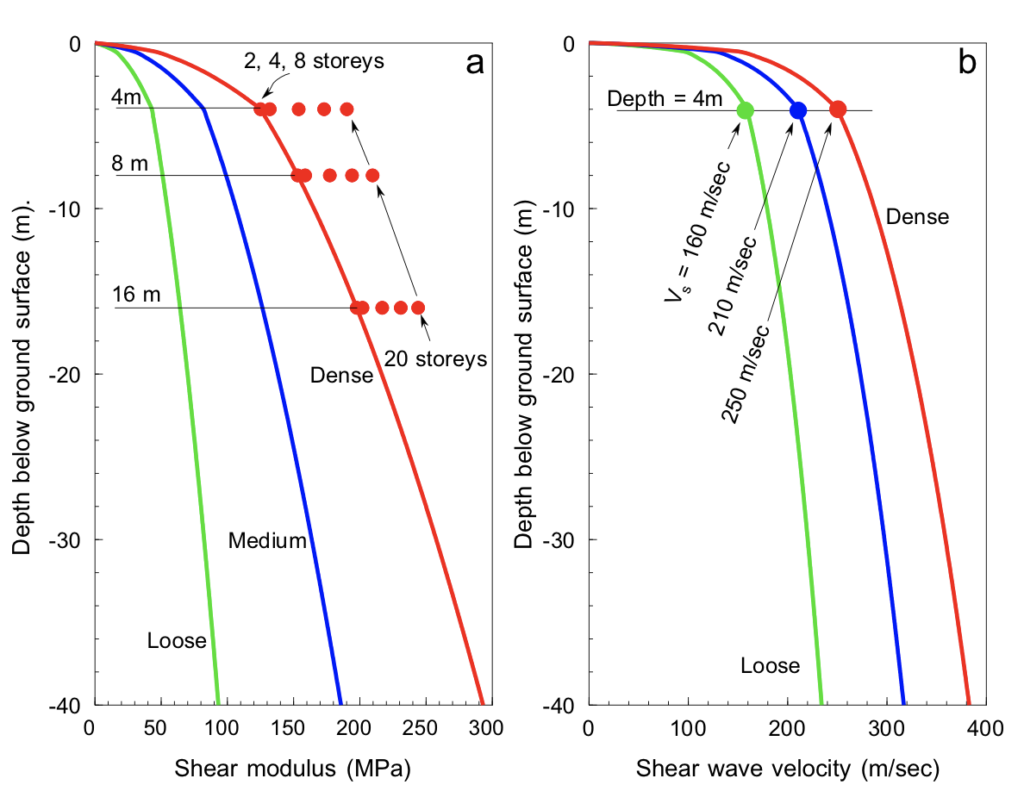Figure 3: Small strain shear modulus and shear wave velocity variation with depth: (a) shear modulus profiles including the contribution from building weight to the dense
profile modulus at depths of 4, 8 and 16 m, (b) level ground shear wave velocity profiles

# 2 SOIL PROFILE

The soil properties are specified with the shear wave velocity and density at a depth of 4 m. The deep sand/gravel layer is assumed to have permeability such that the response of the foundation is drained (that is there is no consideration of liquefaction of the cohesionless soil). The water table is at a depth of 4 m below the ground surface. Three sets of values are used to represent loose, medium, and dense soils. Property values are shown in Table 1 for a depth 4 m beneath the ground surface. Having the shear wave velocity and density at a depth of 4 m the small strain shear modulus is obtained from Vs2. It is well known that soil moduli are a function of the square root of mean effective stress, Ishihara (1995). Thus, the shear wave velocity is a function of the fourth root of the mean effective stress. As the effective stress increases with depth below the ground surface, the shear wave velocity and small strain shear modulus will also increase with depth. The calculated distributions, anchored to the shear wave velocity values at a depth of 4 m for the loose, medium, and dense soil deposits, are shown in Figure 3b.

# 3 FOUNDATION AND STRUCTURE STIFFNESS VALUES

Referring to Figure 2 and equation 2, it is apparent that we need the fixed base natural frequency of each structure (s), the flexural stiffness of column supporting the mass (Ks), the lateral stiffness of the foundation (Kh), and the rotational stiffness of the foundation (K). Once we have these we can use equation 2 to calculate the natural frequency and from there the natural period of the elastic structure-foundation system.

First, the mass of the structures is obtained by assuming a uniform floor loading of 8 kPa (includes permanent and imposed loads).

Second, an equation which gives s comes from the commentary to NZS1170.5 (SANZ 2005) where relationships are given for a range of structural types, all of which are a function of the height of the building. For the calculations discussed herein the period for eccentrically braced steel frame structures is used (1170.5 also gives similar equations for other structural forms):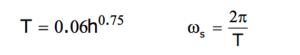(3)

where: T is the fixed base structural period (seconds), and h the height of the structure (metres).

Third, the lateral and rotational stiffnesses of the foundation are required. The square root modulus distributions in Figure 3a apply for the soil beneath a horizontal ground surface. The soil beneath the building foundation has additional stresses due to the weight of the structure so the soil modulus distribution will be complicated, varying both with depth and lateral extent. Herein one point beneath the foundation is used to give a representative value of the modulus of the soil for the whole foundation. Schmertmann (1970) proposed that during settlement of a shallow foundation on sand the maximum vertical strain occurs at a depth of half the foundation width. Burland and Burbidge (1985) found that the depth of influence for the settlement of shallow foundations on sand and gravel is a little more than half the foundation width. Using these ideas as guidance, it was decided to use the soil modulus at a depth of half the foundation width beneath the centre of the foundation as a representative value when estimating the foundation horizontal and rotational stiffnesses – this is the reason for the horizontal lines marking depths of 4, 8 and 16 m in Figure 3a. Additionally it is necessary to allow for the increase in vertical stress in the soil beneath the foundation because of the weight of the buildings. Assuming that the weight of the building is applied to the foundation as a uniform vertical pressure, the increase in vertical stress beneath the centre of a square foundation at a depth of half the width is very close to the applied pressure, Harr (1966). With this information the representative soil modulus beneath the foundation can be updated and it is now a function of the number of building storeys, as shown in Figure 3a for the dense profile (similar behaviour was calculated for the loose and medium profiles). The lateral and rotational stiffnesses of the foundation were calculated using the equations given by Gazetas (1991) which account for the shape of the foundation and the effect of embedment. Similar stiffness expressions are given in the NIST (2012) document.

# 4 RESULTS

Having the stiffness values for the structures and the foundations we can estimate what benefits will be obtained from SSI effects. The first step is to use equation (2) to obtain the period of the structure-foundation system incorporating SSI effects. Next the Spectral Shape Factor curves in NZS1170.5:2004 (SANZ 2004) are used to get comparative values of the earthquake design spectral accelerations (these curves are associated with a notional damping coefficient value of 5%). The ordinate in the NZS1170.5 curves gives a value which is multiplied by a hazard factor, a return period factor, and a near-fault factor to give the earthquake design spectral acceleration at a specific site location. But, for the work reported in this paper, comparing values for the spectral shape factor is adequate. Referring to Figure 1, comparison of the points marked A and B is used to obtain the SSI period lengthening and the reduction in the spectral shape factor. These changes are normalised with respect to the values associated with point A. Figure 4 shows the period elongation, expressed as a ratio of the value at point A, in moving from point A to B in Figure 1. Figure 5 has a similar plot, but this time the ratio given is that of the spectral shape coefficient values for the NZS 1170.5 Shallow ground curve. In both Figures 4 and 5 there is a reduction in the spectral shape coefficient because the fixed base structural period is positioned along the falling branch of the spectral shape coefficient curve.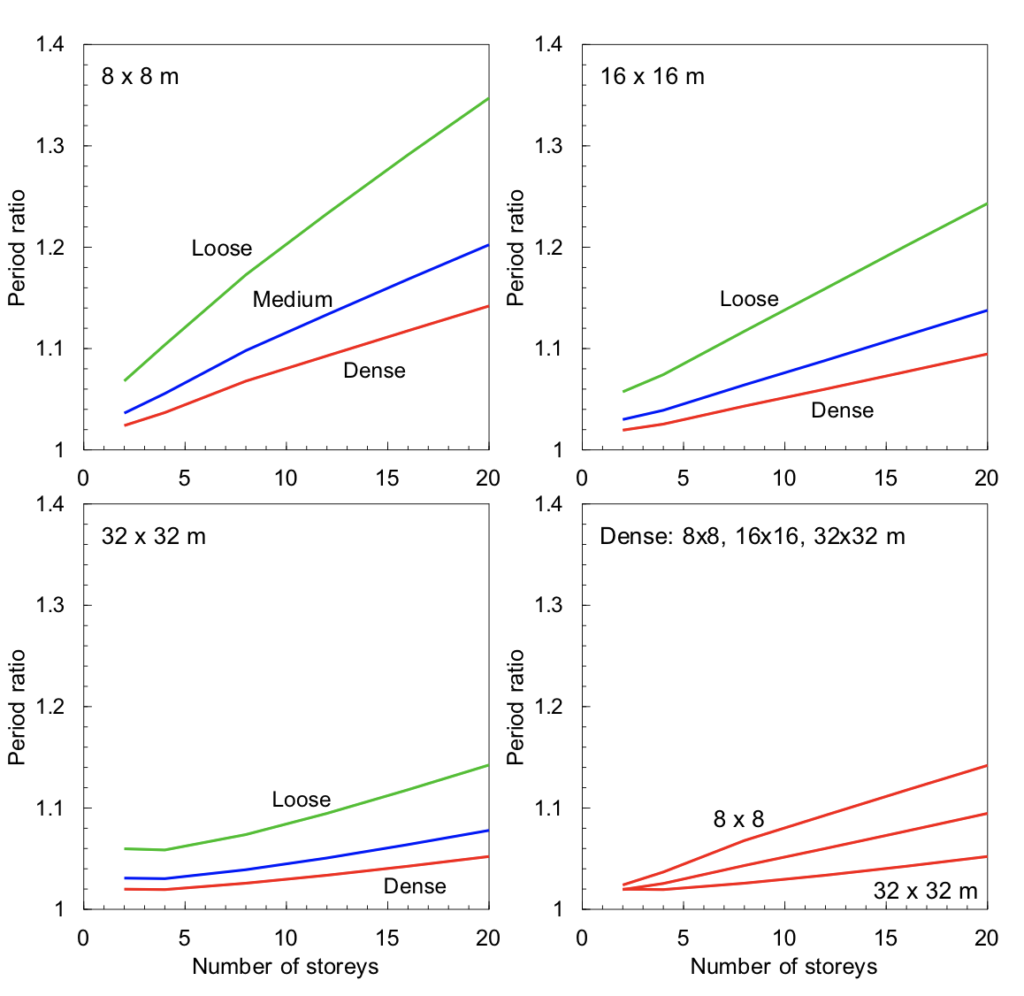Figure 4: SSI induced period lengthening, expressed as a ratio of the SSI period to the fixed base period, for the various building configurations and ground conditions

# 5 DISCUSSION

Unsurprisingly the various parts of Figures 4 and 5 show that the loose soil deposit (Vs = 160 m/sec) has the greatest period elongation and reduction in spectral shape coefficient. It is apparent that, for the medium and dense soil conditions, the period lengthening is significantly less. Again, unsurprisingly, the smallest foundation demonstrates the largest SSI modifications to response.

We will limit our considerations to the buildings with 16 and 32 m square plan dimensions as an 8 m square tower 20 storeys in height is not a serious building configuration (but might be part of a guyed chimney or radio mast). A 32 m square tower would be representative of many typical buildings and 16 m square tower perhaps representative of the slim end of the range of typical structures. Furthermore, it is unlikely that a shallow foundation on loose sand would be considered for a tall building. Thus we are left with 16 and 32 m square building plan dimensions with shallow foundations on medium and dense soil. For these configurations Figures 4 and 5 show that even for the tallest buildings, elastic soil-structure interaction has only a minor contribution to the performance of the structure-foundation system.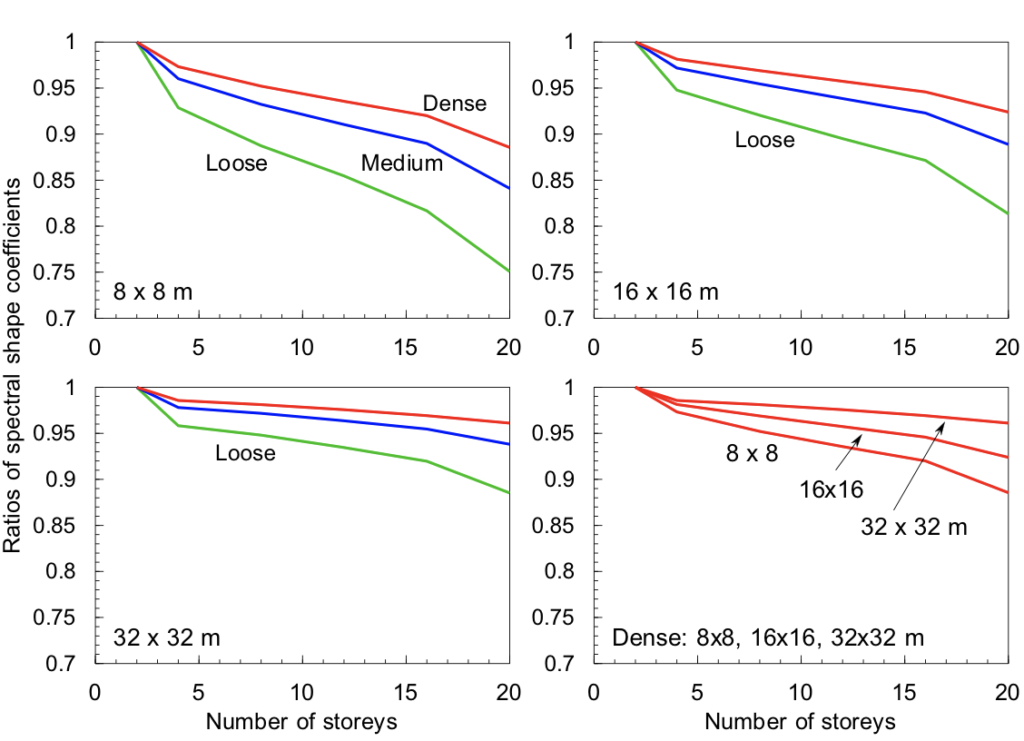Figure 5: SSI induced reduction in the spectral shape coefficient, expressed as a ratio spectral shape coefficients from NZS1170.5 shallow site condition, for the various buildingconfigurations and ground conditions

Figures 4 and 5 were derived for the Shallow soil spectral shape coefficient curve in NZS1170.5, similar conclusions are reached when the Deep soil curve is used.

However, it is expected that soil beneath a shallow foundation will not remain elastic during a design earthquake and nonlinear deformation will take place with an effective reduction in the soil modulus. Table 4.1 in Part V of Eurocode 8 (BSI 2005) gives suggestions for this reduction with respect to the earthquake peak ground acceleration. For a PGA of about 0.3g it is suggested that an equivalent modulus about one third of the small strain value be used. Referring to Table 1 it is apparent that such a reduction would reduce the small strain stiffness of the dense soil to about the value for the loose material. Looking again at the lines for loose behaviour in Figures 4 and 5 it is apparent that nonlinear soil behaviour might provide a 15 to 20% reduction in foundation actions when tall 16 and 32 m square structures are subject to a modest earthquake.

Evaluating, and plotting against number of storeys, the values for the three dimensionless parameters given in equation (1), no additional insight is obtained. The values of all three parameters increase as the number of storeys increases. The structures with the 8 m square plan dimension with foundations on loose soil having the largest values for all three parameters, and the 32 m square plan dimension buildings with foundations on dense soil having the smallest values.

An important factor not considered above is the effect of damping on soil-structure interaction. Increasing damping, caused by hysteretic soil deformation, will move the design spectrum in Figure 1 downwards, so providing an additional mechanism to reduce design actions. Elastic radiation damping is small for foundation rotational deformation modes, the main foundation deformation mechanism for tall structures on shallow foundations, and so nonlinear soil deformation is important. Part V of Eurocode suggests a hysteretic damping coefficient value of about 10% when the ground around the foundation is subject to a PGA of about 0.3g. Recent work on nonlinear moment-rotation behaviour of shallow foundations illustrates how rocking (that is cyclic loss of contact at the foundation edges during the earthquake loading) further reduces foundation actions (Pender el al. 2017a and b).

# 6 CONCLUSIONS

The calculations reported herein show that elastic soil-structure interaction is of negligible benefit for buildings on shallow foundations in medium and dense cohesionless soils, Figures 4 and 5. Thus the answer to the rhetorical question at the end of the title of this paper is negative.

To achieve a soil-structure interaction related improvement in building performance during earthquakes, nonlinear behaviour of the zone of soil around and beneath the foundation is needed to reduce the stiffness of the soil and provide hysteretic damping.

Part V of Eurocode 8 provides guidance for reducing soil stiffness and increasing hysteretic damping as earthquake peak ground acceleration increases. This provides an approximate simplified way to handle the complexities of the nonlinear cyclic stress-strain behaviour of soil.

# REFERENCES

BSI (2005). BS EN 1998-1:2005: Eurocode 8: Design of structures for earthquake resistance – Part V: Foundations, retaining structures and geotechnical aspects. British Standards Institution, London, UK.

Burland, J. B. and Burbidge, M. C. (1985). Settlement of foundations on sand and gravel. Proc. ICE, Part 1, pp. 1325‑1381.

Chopra, A. K. (1995). Dynamics of Structures. Prentice-Hall, New Jersey.

Gazetas, G. (1991). Foundation vibrations, in Foundation Engineering Handbook, 2nd. edition, H-Y Fang editor, Van Nostrand Reinhold, pp. 553-593.

Harr, M. E. (1966). Foundations of theoretical soil mechanics. MGraw-Hill, New York.

Ishihara, K. (!996). Soil behaviour in earthquake geotechnics. Oxford Engineering Science, Oxford, UK.

NIST (2012). Soil-structure interaction for building structures. NIST GCR 12-917-21, National Institute of Standards and Technology, Gaithersberg, Maryland.

Pender, M. J., Algie, T. B., Storie, L. B. and Salimath, R. (2017a). One dimensional moment-rotation macro-element for performance based design of shallow foundations. Proceedings Performance Based Design III (PBDIII), Vancouver.

Pender, M. J., Algie, T. B., Storie, L. B. and Salimath, R. (2017b). One dimensional shallow foundation macro-element. In: Developments in Earthquake Geotechnics, S. Iai ed. Springer, Singapore.

SANZ (2004).NZS 1170.5: 2004. Structural Design Actions Part 5: Earthquake Actions – New Zealand. Standards New Zealand, Wellington.

Schmertmann, J. H. (1970). Static cone to compute static settlement over sand. Proc. ASCE, Jnl. Soil Mechanics Div., Vol. 96 SM3, pp. 1011‑1043.

Wolf, J. P. (1985). Dynamic Soil-Structure Interaction. Prentice Hall, New Jersey.NZGS_Symposium_20_Pender1 (528 KB)